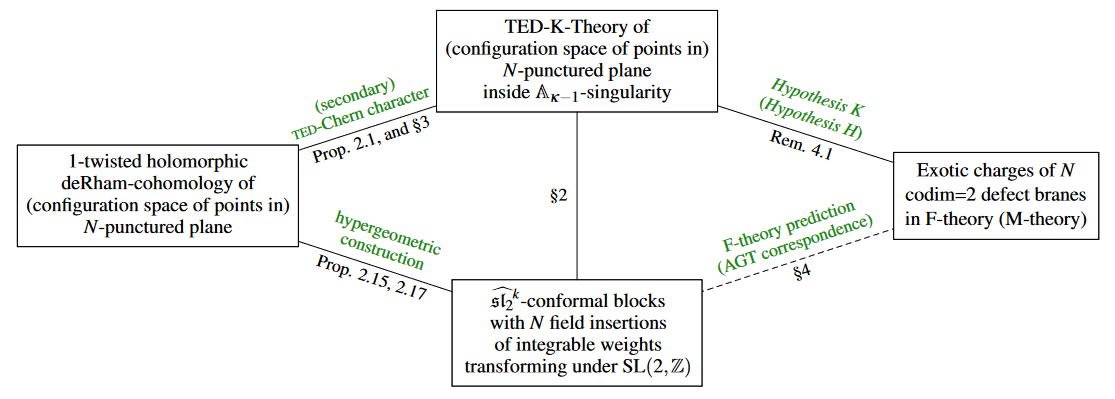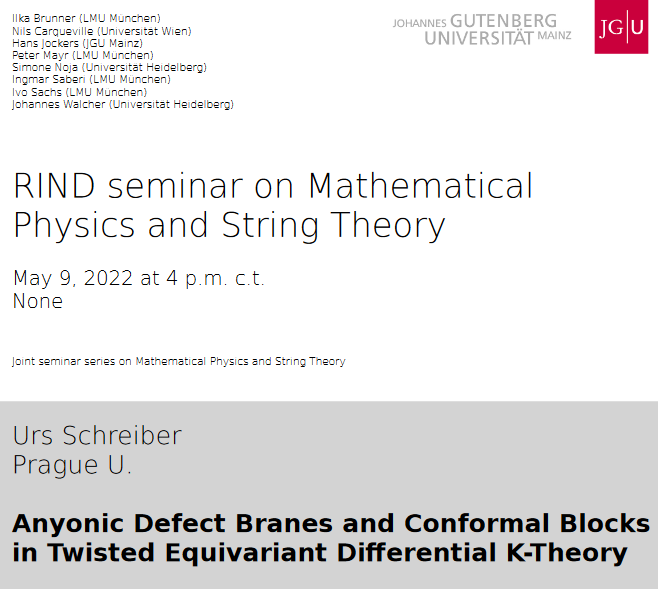# Schreiber Anyonic defect branes in TED-K-theory

An article that we have written:

• Hisham Sati$\,$ and $\,$ Urs Schreiber:

Anyonic Defect Branes and Conformal Blocks in

Twisted Equivariant Differential (TED) K-Theory

Reviews in Mathematical Physics (2023, in print)

Abstract: We demonstrate that twisted equivariant differential K-theory of transverse complex curves accommodates exotic charges of the form expected of codimension=2 defect branes, such as of D7-branes in IIB/F-theory on $\mathbb{A}$-type orbifold singularities, but also of their dual 3-brane defects of class-S theories on M5-branes. These branes have been argued, within F-theory and the AGT correspondence, to carry special $\mathrm{SL}(2)$-monodromy charges not seen for other branes, at least partially reflected in conformal blocks of the $\widehat{\mathfrak{sl}_2}$-WZW model over their transverse punctured complex curve. Indeed, it has been argued that all "exotic" branes of string theory are defect branes carrying such U-duality monodromy charges – but none of these had previously been identified in the expected brane charge quantization law given by K-theory.

Here we observe that it is the subtle (and previously somewhat neglected) twisting of equivariant K-theory by flat complex line bundles appearing inside orbi-singularities (“inner local systems”) that makes the secondary Chern character on a punctured plane inside an $\mathbb{A}$-type singularity evaluate to the twisted holomorphic de Rham cohomology which Feigin, Schechtman and Varchenko showed realizes $\widehat{\mathfrak{sl}_2}$-conformal blocks, here in degree 1 – in fact it gives the direct sum of these over all admissible fractional levels $k = - 2 + \kappa/r$. The remaining higher-degree $\widehat{\mathfrak{sl}_2}$-conformal blocks appear similarly if we assume our previously discussed “Hypothesis H” about brane charge quantization in M-theory. Since conformal blocks – and hence these twisted equivariant secondary Chern characters – solve the Knizhnik-Zamolodchikov equation and thus constitute representations of the braid group of motions of defect branes inside their transverse space, this provides a concrete first-principles realization of anyon statistics of – and hence of topological quantum computation on – defect branes in string/M-theory.Related articles:

For more see at Hypothesis H.

Expository talk:Related talks:

Last revised on January 10, 2023 at 09:15:13. See the history of this page for a list of all contributions to it.